Main MRPT website > C++ reference for MRPT 1.5.7
mrpt::poses::CPosePDFGaussianInf Class Referenceabstract

## Detailed Description

A Probability Density function (PDF) of a 2D pose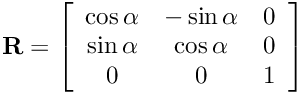as a Gaussian with a mean and the inverse of the covariance.

This class implements a PDF as a mono-modal Gaussian distribution in its information form, that is, keeping the inverse of the covariance matrix instead of the covariance matrix itself.

This class is the dual of CPosePDFGaussian.

CPose2D, CPosePDF, CPosePDFParticles

Definition at line 35 of file CPosePDFGaussianInf.h.

#include <mrpt/poses/CPosePDFGaussianInf.h>

Inheritance diagram for mrpt::poses::CPosePDFGaussianInf: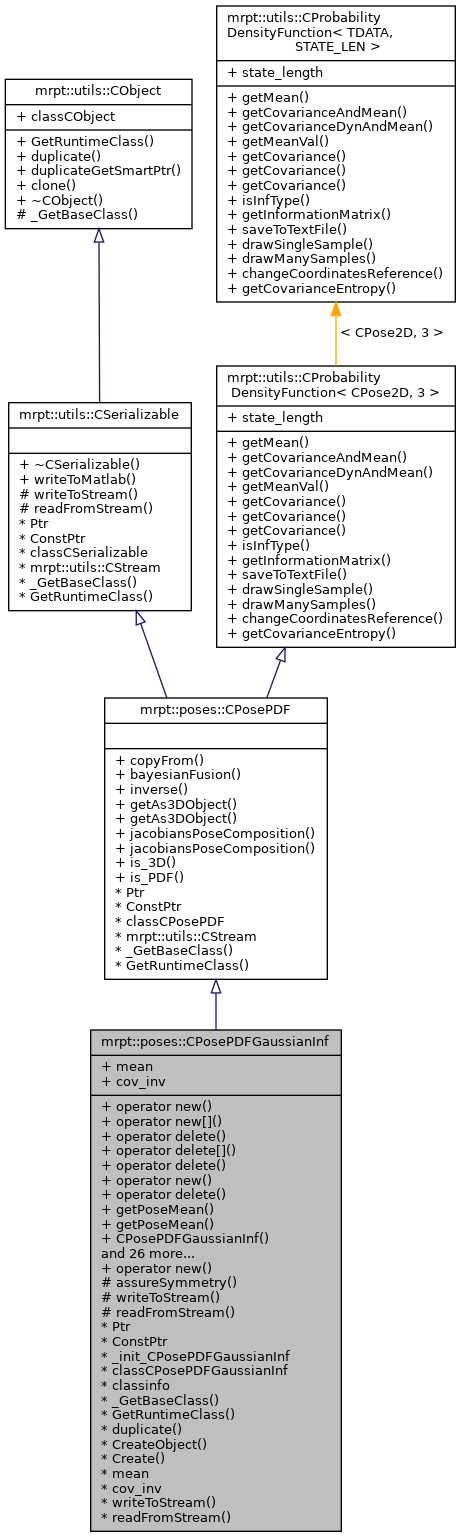## Public Types

enum  { is_3D_val = 0 }

enum  { is_PDF_val = 1 }

typedef CPose2D type_value
The type of the state the PDF represents. More...

## Public Member Functions

voidoperator new (size_t size)

voidoperator new[] (size_t size)

void operator delete (void *ptr) throw ()

void operator delete[] (void *ptr) throw ()

void operator delete (void *memory, void *ptr) throw ()

voidoperator new (size_t size, const std::nothrow_t &) throw ()

void operator delete (void *ptr, const std::nothrow_t &) throw ()

const CPose2DgetPoseMean () const

CPose2DgetPoseMean ()

CPosePDFGaussianInf ()
Default constructor (mean=all zeros, inverse covariance=all zeros -> so be careful!) More...

CPosePDFGaussianInf (const CPose2D &init_Mean)
Constructor with a mean value (inverse covariance=all zeros -> so be careful!) More...

CPosePDFGaussianInf (const CPose2D &init_Mean, const mrpt::math::CMatrixDouble33 &init_CovInv)
Constructor. More...

CPosePDFGaussianInf (const CPosePDF &o)
Copy constructor, including transformations between other PDFs. More...

CPosePDFGaussianInf (const CPose3DPDF &o)
Copy constructor, including transformations between other PDFs. More...

void getMean (CPose2D &mean_pose) const MRPT_OVERRIDE
Returns an estimate of the pose, (the mean, or mathematical expectation of the PDF). More...

bool isInfType () const MRPT_OVERRIDE
Returns whether the class instance holds the uncertainty in covariance or information form. More...

void getCovarianceAndMean (mrpt::math::CMatrixDouble33 &cov, CPose2D &mean_point) const MRPT_OVERRIDE
Returns an estimate of the pose covariance matrix (3x3 cov matrix) and the mean, both at once. More...

virtual void getInformationMatrix (mrpt::math::CMatrixDouble33 &inf) const MRPT_OVERRIDE
Returns the information (inverse covariance) matrix (a STATE_LEN x STATE_LEN matrix) More...

void copyFrom (const CPosePDF &o) MRPT_OVERRIDE
Copy operator, translating if necesary (for example, between particles and gaussian representations) More...

void copyFrom (const CPose3DPDF &o)
Copy operator, translating if necesary (for example, between particles and gaussian representations) More...

void saveToTextFile (const std::string &file) const MRPT_OVERRIDE
Save PDF's particles to a text file, containing the 2D pose in the first line, then the covariance matrix in next 3 lines. More...

void changeCoordinatesReference (const CPose3D &newReferenceBase) MRPT_OVERRIDE
this = p (+) this. More...

void changeCoordinatesReference (const CPose2D &newReferenceBase)
this = p (+) this. More...

void rotateCov (const double ang)
Rotate the covariance matrix by replacing it by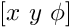, where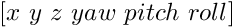. More...

void inverseComposition (const CPosePDFGaussianInf &x, const CPosePDFGaussianInf &ref)
Set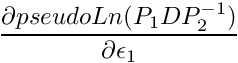, computing the mean using the "-" operator and the covariances through the corresponding Jacobians (For 'x0' and 'x1' being independent variables!). More...

void inverseComposition (const CPosePDFGaussianInf &x1, const CPosePDFGaussianInf &x0, const mrpt::math::CMatrixDouble33 &COV_01)
Set, computing the mean using the "-" operator and the covariances through the corresponding Jacobians (Given the 3x3 cross-covariance matrix of variables x0 and x1). More...

void drawSingleSample (CPose2D &outPart) const MRPT_OVERRIDE
Draws a single sample from the distribution. More...

void drawManySamples (size_t N, std::vector< mrpt::math::CVectorDouble > &outSamples) const MRPT_OVERRIDE
Draws a number of samples from the distribution, and saves as a list of 1x3 vectors, where each row contains a (x,y,phi) datum. More...

void bayesianFusion (const CPosePDF &p1, const CPosePDF &p2, const double &minMahalanobisDistToDrop=0) MRPT_OVERRIDE
Bayesian fusion of two points gauss. More...

void inverse (CPosePDF &o) const MRPT_OVERRIDE
Returns a new PDF such as: NEW_PDF = (0,0,0) - THIS_PDF. More...

void operator+= (const CPose2D &Ap)
Makes: thisPDF = thisPDF + Ap, where "+" is pose composition (both the mean, and the covariance matrix are updated). More...

double evaluatePDF (const CPose2D &x) const
Evaluates the PDF at a given point. More...

double evaluateNormalizedPDF (const CPose2D &x) const
Evaluates the ratio PDF(x) / PDF(MEAN), that is, the normalized PDF in the range [0,1]. More...

double mahalanobisDistanceTo (const CPosePDFGaussianInf &theOther)
Computes the Mahalanobis distance between the centers of two Gaussians. More...

void operator+= (const CPosePDFGaussianInf &Ap)
Makes: thisPDF = thisPDF + Ap, where "+" is pose composition (both the mean, and the covariance matrix are updated) (see formulas in jacobiansPoseComposition ). More...

void operator-= (const CPosePDFGaussianInf &ref)
Makes: thisPDF = thisPDF - Ap, where "-" is pose inverse composition (both the mean, and the covariance matrix are updated) More...

template<class OPENGL_SETOFOBJECTSPTR >
void getAs3DObject (OPENGL_SETOFOBJECTSPTR &out_obj) const
Returns a 3D representation of this PDF (it doesn't clear the current contents of out_obj, but append new OpenGL objects to that list) More...

template<class OPENGL_SETOFOBJECTSPTR >
OPENGL_SETOFOBJECTSPTR getAs3DObject () const
Returns a 3D representation of this PDF. More...

virtual mxArraywriteToMatlab () const
Introduces a pure virtual method responsible for writing to a mxArray Matlab object, typically a MATLAB struct whose contents are documented in each derived class. More...

mrpt::utils::CObjectPtr duplicateGetSmartPtr () const
Returns a copy of the object, indepently of its class, as a smart pointer (the newly created object will exist as long as any copy of this smart pointer). More...

CObjectclone () const
Cloning interface for smart pointers. More...

virtual void getMean (CPose2D &mean_point) const=0
Returns the mean, or mathematical expectation of the probability density distribution (PDF). More...

virtual void getCovarianceAndMean (mrpt::math::CMatrixFixedNumeric< double, STATE_LEN, STATE_LEN > &cov, CPose2D &mean_point) const=0
Returns an estimate of the pose covariance matrix (STATE_LENxSTATE_LEN cov matrix) and the mean, both at once. More...

void getCovarianceDynAndMean (mrpt::math::CMatrixDouble &cov, CPose2D &mean_point) const
Returns an estimate of the pose covariance matrix (STATE_LENxSTATE_LEN cov matrix) and the mean, both at once. More...

CPose2D getMeanVal () const
Returns the mean, or mathematical expectation of the probability density distribution (PDF). More...

void getCovariance (mrpt::math::CMatrixDouble &cov) const
Returns the estimate of the covariance matrix (STATE_LEN x STATE_LEN covariance matrix) More...

void getCovariance (mrpt::math::CMatrixFixedNumeric< double, STATE_LEN, STATE_LEN > &cov) const
Returns the estimate of the covariance matrix (STATE_LEN x STATE_LEN covariance matrix) More...

mrpt::math::CMatrixFixedNumeric< double, STATE_LEN, STATE_LEN > getCovariance () const
Returns the estimate of the covariance matrix (STATE_LEN x STATE_LEN covariance matrix) More...

virtual void getInformationMatrix (mrpt::math::CMatrixFixedNumeric< double, STATE_LEN, STATE_LEN > &inf) const
Returns the information (inverse covariance) matrix (a STATE_LEN x STATE_LEN matrix) Unless reimplemented in derived classes, this method first reads the covariance, then invert it. More...

virtual void drawSingleSample (CPose2D &outPart) const=0
Draws a single sample from the distribution. More...

double getCovarianceEntropy () const
Compute the entropy of the estimated covariance matrix. More...

## Static Public Member Functions

static voidoperator new (size_t size, void *ptr)

static void jacobiansPoseComposition (const CPose2D &x, const CPose2D &u, mrpt::math::CMatrixDouble33 &df_dx, mrpt::math::CMatrixDouble33 &df_du, const bool compute_df_dx=true, const bool compute_df_du=true)
This static method computes the pose composition Jacobians, with these formulas: More...

static void jacobiansPoseComposition (const CPosePDFGaussian &x, const CPosePDFGaussian &u, mrpt::math::CMatrixDouble33 &df_dx, mrpt::math::CMatrixDouble33 &df_du)

static bool is_3D ()

static bool is_PDF ()

## Public Attributes

Data fields
CPose2D mean
The mean value. More...

mrpt::math::CMatrixDouble33 cov_inv
The inverse of the 3x3 covariance matrix (the "information" matrix) More...

## Static Public Attributes

static const mrpt::utils::TRuntimeClassId classCObject

static const size_t state_length
The length of the variable, for example, 3 for a 3D point, 6 for a 3D pose (x y z yaw pitch roll). More...

RTTI stuff
static const mrpt::utils::TRuntimeClassId classCPosePDF

RTTI stuff
static const mrpt::utils::TRuntimeClassId classCSerializable

## Protected Types

typedef CPosePDFGaussianInf self_t

## Protected Member Functions

void assureSymmetry ()
Assures the symmetry of the covariance matrix (eventually certain operations in the math-coprocessor lead to non-symmetric matrixes!) More...

CSerializable virtual methods
void writeToStream (mrpt::utils::CStream &out, int *getVersion) const
Introduces a pure virtual method responsible for writing to a CStream. More...

void readFromStream (mrpt::utils::CStream &in, int version)
Introduces a pure virtual method responsible for loading from a CStream This can not be used directly be users, instead use "stream >> object;" for reading it from a stream or "stream >> object_ptr;" if the class is unknown apriori. More...

## RTTI stuff

typedef CPosePDFGaussianInfPtr Ptr

typedef CPosePDFGaussianInfPtr ConstPtr

static mrpt::utils::CLASSINIT _init_CPosePDFGaussianInf

static mrpt::utils::TRuntimeClassId classCPosePDFGaussianInf

static const mrpt::utils::TRuntimeClassIdclassinfo

static const mrpt::utils::TRuntimeClassId_GetBaseClass ()

virtual const mrpt::utils::TRuntimeClassIdGetRuntimeClass () const
Returns information about the class of an object in runtime. More...

virtual mrpt::utils::CObjectduplicate () const
Returns a copy of the object, indepently of its class. More...

static mrpt::utils::CObjectCreateObject ()

static CPosePDFGaussianInfPtr Create ()

## ◆ ConstPtr

 typedef CPosePDFGaussianInfPtr mrpt::poses::CPosePDFGaussianInf::ConstPtr

Definition at line 38 of file CPosePDFGaussianInf.h.

## ◆ Ptr

 typedef CPosePDFGaussianInfPtr mrpt::poses::CPosePDFGaussianInf::Ptr

A typedef for the associated smart pointer

Definition at line 38 of file CPosePDFGaussianInf.h.

## ◆ self_t

 protected

Definition at line 38 of file CPosePDFGaussianInf.h.

## ◆ type_value

 typedef CPose2D mrpt::utils::CProbabilityDensityFunction< CPose2D , STATE_LEN >::type_value
inherited

The type of the state the PDF represents.

Definition at line 32 of file CProbabilityDensityFunction.h.

## ◆ anonymous enum

 anonymous enum
inherited
Enumerator
is_3D_val

Definition at line 91 of file CPosePDF.h.

## ◆ anonymous enum

 anonymous enum
inherited
Enumerator
is_PDF_val

Definition at line 93 of file CPosePDF.h.

## ◆ CPosePDFGaussianInf() [1/5]

 CPosePDFGaussianInf::CPosePDFGaussianInf ( )

Default constructor (mean=all zeros, inverse covariance=all zeros -> so be careful!)

Definition at line 40 of file CPosePDFGaussianInf.cpp.

## ◆ CPosePDFGaussianInf() [2/5]

 CPosePDFGaussianInf::CPosePDFGaussianInf ( const CPose2D & init_Mean )
explicit

Constructor with a mean value (inverse covariance=all zeros -> so be careful!)

Definition at line 56 of file CPosePDFGaussianInf.cpp.

## ◆ CPosePDFGaussianInf() [3/5]

 CPosePDFGaussianInf::CPosePDFGaussianInf ( const CPose2D & init_Mean, const mrpt::math::CMatrixDouble33 & init_CovInv )

Constructor.

Definition at line 47 of file CPosePDFGaussianInf.cpp.

## ◆ CPosePDFGaussianInf() [4/5]

 mrpt::poses::CPosePDFGaussianInf::CPosePDFGaussianInf ( const CPosePDF & o )
inlineexplicit

Copy constructor, including transformations between other PDFs.

Definition at line 68 of file CPosePDFGaussianInf.h.

## ◆ CPosePDFGaussianInf() [5/5]

 mrpt::poses::CPosePDFGaussianInf::CPosePDFGaussianInf ( const CPose3DPDF & o )
inlineexplicit

Copy constructor, including transformations between other PDFs.

Definition at line 71 of file CPosePDFGaussianInf.h.

## ◆ _GetBaseClass()

 static const mrpt::utils::TRuntimeClassId* mrpt::poses::CPosePDFGaussianInf::_GetBaseClass ( )
staticprotected

## ◆ assureSymmetry()

 void CPosePDFGaussianInf::assureSymmetry ( )
protected

Assures the symmetry of the covariance matrix (eventually certain operations in the math-coprocessor lead to non-symmetric matrixes!)

Definition at line 377 of file CPosePDFGaussianInf.cpp.

References cov_inv.

## ◆ bayesianFusion()

 void CPosePDFGaussianInf::bayesianFusion ( const CPosePDF & p1, const CPosePDF & p2, const double & minMahalanobisDistToDrop = 0 )
virtual

Bayesian fusion of two points gauss.

distributions, then save the result in this object. The process is as follows:

• (x1,S1): Mean and variance of the p1 distribution.
• (x2,S2): Mean and variance of the p2 distribution.
• (x,S): Mean and variance of the resulting distribution.

S = (S1-1 + S2-1)-1; x = S * ( S1-1*x1 + S2-1*x2 );

Implements mrpt::poses::CPosePDF.

Definition at line 279 of file CPosePDFGaussianInf.cpp.

## ◆ changeCoordinatesReference() [1/2]

 void CPosePDFGaussianInf::changeCoordinatesReference ( const CPose3D & newReferenceBase )
virtual

this = p (+) this.

This can be used to convert a PDF from local coordinates to global, providing the point (newReferenceBase) from which "to project" the current pdf. Result PDF substituted the currently stored one in the object

Definition at line 176 of file CPosePDFGaussianInf.cpp.

Referenced by operator+().

## ◆ changeCoordinatesReference() [2/2]

 void CPosePDFGaussianInf::changeCoordinatesReference ( const CPose2D & newReferenceBase )

this = p (+) this.

This can be used to convert a PDF from local coordinates to global, providing the point (newReferenceBase) from which "to project" the current pdf. Result PDF substituted the currently stored one in the object.

Definition at line 190 of file CPosePDFGaussianInf.cpp.

## ◆ clone()

 CObject* mrpt::utils::CObject::clone ( ) const
inlineinherited

Cloning interface for smart pointers.

Definition at line 143 of file CObject.h.

## ◆ copyFrom() [1/2]

 void CPosePDFGaussianInf::copyFrom ( const CPosePDF & o )
virtual

Copy operator, translating if necesary (for example, between particles and gaussian representations)

Implements mrpt::poses::CPosePDF.

Definition at line 100 of file CPosePDFGaussianInf.cpp.

## ◆ copyFrom() [2/2]

 void CPosePDFGaussianInf::copyFrom ( const CPose3DPDF & o )

Copy operator, translating if necesary (for example, between particles and gaussian representations)

Definition at line 123 of file CPosePDFGaussianInf.cpp.

## ◆ Create()

 static CPosePDFGaussianInfPtr mrpt::poses::CPosePDFGaussianInf::Create ( )
static

## ◆ CreateObject()

 static mrpt::utils::CObject* mrpt::poses::CPosePDFGaussianInf::CreateObject ( )
static

## ◆ drawManySamples()

 void CPosePDFGaussianInf::drawManySamples ( size_t N, std::vector< mrpt::math::CVectorDouble > & outSamples ) const
virtual

Draws a number of samples from the distribution, and saves as a list of 1x3 vectors, where each row contains a (x,y,phi) datum.

Reimplemented from mrpt::utils::CProbabilityDensityFunction< CPose2D, 3 >.

Definition at line 249 of file CPosePDFGaussianInf.cpp.

## ◆ drawSingleSample() [1/2]

 virtual void mrpt::utils::CProbabilityDensityFunction< CPose2D , STATE_LEN >::drawSingleSample ( CPose2D & outPart ) const
pure virtualinherited

Draws a single sample from the distribution.

## ◆ drawSingleSample() [2/2]

 void CPosePDFGaussianInf::drawSingleSample ( CPose2D & outPart ) const

Draws a single sample from the distribution.

Definition at line 224 of file CPosePDFGaussianInf.cpp.

## ◆ duplicate()

 virtual mrpt::utils::CObject* mrpt::poses::CPosePDFGaussianInf::duplicate ( ) const
virtual

Returns a copy of the object, indepently of its class.

Implements mrpt::utils::CObject.

## ◆ duplicateGetSmartPtr()

 mrpt::utils::CObjectPtr mrpt::utils::CObject::duplicateGetSmartPtr ( ) const
inlineinherited

Returns a copy of the object, indepently of its class, as a smart pointer (the newly created object will exist as long as any copy of this smart pointer).

Definition at line 140 of file CObject.h.

## ◆ evaluateNormalizedPDF()

 double CPosePDFGaussianInf::evaluateNormalizedPDF ( const CPose2D & x ) const

Evaluates the ratio PDF(x) / PDF(MEAN), that is, the normalized PDF in the range [0,1].

Definition at line 363 of file CPosePDFGaussianInf.cpp.

## ◆ evaluatePDF()

 double CPosePDFGaussianInf::evaluatePDF ( const CPose2D & x ) const

Evaluates the PDF at a given point.

Definition at line 352 of file CPosePDFGaussianInf.cpp.

References cov_inv, mean, and mrpt::math::normalPDF().

## ◆ getAs3DObject() [1/2]

template<class OPENGL_SETOFOBJECTSPTR >
 void mrpt::poses::CPosePDF::getAs3DObject ( OPENGL_SETOFOBJECTSPTR & out_obj ) const
inlineinherited

Returns a 3D representation of this PDF (it doesn't clear the current contents of out_obj, but append new OpenGL objects to that list)

Note
Needs the mrpt-opengl library, and using mrpt::opengl::CSetOfObjectsPtr as template argument.
By default, ellipsoids for the confidence intervals of "q=3" are drawn; for more mathematical details, see CGeneralizedEllipsoidTemplate::setQuantiles()

Definition at line 101 of file CPosePDF.h.

References mrpt::opengl::posePDF2opengl().

## ◆ getAs3DObject() [2/2]

template<class OPENGL_SETOFOBJECTSPTR >
 OPENGL_SETOFOBJECTSPTR mrpt::poses::CPosePDF::getAs3DObject ( ) const
inlineinherited

Returns a 3D representation of this PDF.

Note
Needs the mrpt-opengl library, and using mrpt::opengl::CSetOfObjectsPtr as template argument.

Definition at line 110 of file CPosePDF.h.

References mrpt::opengl::posePDF2opengl().

## ◆ getCovariance() [1/3]

 void mrpt::utils::CProbabilityDensityFunction< CPose2D , STATE_LEN >::getCovariance ( mrpt::math::CMatrixDouble & cov ) const
inlineinherited

Returns the estimate of the covariance matrix (STATE_LEN x STATE_LEN covariance matrix)

getMean, getCovarianceAndMean, getInformationMatrix

Definition at line 68 of file CProbabilityDensityFunction.h.

## ◆ getCovariance() [2/3]

 void mrpt::utils::CProbabilityDensityFunction< CPose2D , STATE_LEN >::getCovariance ( mrpt::math::CMatrixFixedNumeric< double, STATE_LEN, STATE_LEN > & cov ) const
inlineinherited

Returns the estimate of the covariance matrix (STATE_LEN x STATE_LEN covariance matrix)

getMean, getCovarianceAndMean, getInformationMatrix

Definition at line 77 of file CProbabilityDensityFunction.h.

## ◆ getCovariance() [3/3]

 mrpt::math::CMatrixFixedNumeric mrpt::utils::CProbabilityDensityFunction< CPose2D , STATE_LEN >::getCovariance ( ) const
inlineinherited

Returns the estimate of the covariance matrix (STATE_LEN x STATE_LEN covariance matrix)

getMean, getInformationMatrix

Definition at line 86 of file CProbabilityDensityFunction.h.

## ◆ getCovarianceAndMean() [1/2]

 virtual void mrpt::utils::CProbabilityDensityFunction< CPose2D , STATE_LEN >::getCovarianceAndMean ( mrpt::math::CMatrixFixedNumeric< double, STATE_LEN, STATE_LEN > & cov, CPose2D & mean_point ) const
pure virtualinherited

Returns an estimate of the pose covariance matrix (STATE_LENxSTATE_LEN cov matrix) and the mean, both at once.

getMean, getInformationMatrix

## ◆ getCovarianceAndMean() [2/2]

 void mrpt::poses::CPosePDFGaussianInf::getCovarianceAndMean ( mrpt::math::CMatrixDouble33 & cov, CPose2D & mean_point ) const
inline

Returns an estimate of the pose covariance matrix (3x3 cov matrix) and the mean, both at once.

getMean

Definition at line 82 of file CPosePDFGaussianInf.h.

References mrpt::math::cov(), and mean().

## ◆ getCovarianceDynAndMean()

 void mrpt::utils::CProbabilityDensityFunction< CPose2D , STATE_LEN >::getCovarianceDynAndMean ( mrpt::math::CMatrixDouble & cov, CPose2D & mean_point ) const
inlineinherited

Returns an estimate of the pose covariance matrix (STATE_LENxSTATE_LEN cov matrix) and the mean, both at once.

getMean, getInformationMatrix

Definition at line 48 of file CProbabilityDensityFunction.h.

## ◆ getCovarianceEntropy()

 double mrpt::utils::CProbabilityDensityFunction< CPose2D , STATE_LEN >::getCovarianceEntropy ( ) const
inlineinherited

Compute the entropy of the estimated covariance matrix.

http://en.wikipedia.org/wiki/Multivariate_normal_distribution#Entropy

Definition at line 144 of file CProbabilityDensityFunction.h.

## ◆ getInformationMatrix() [1/2]

 virtual void mrpt::poses::CPosePDFGaussianInf::getInformationMatrix ( mrpt::math::CMatrixDouble33 & inf ) const
inlinevirtual

Returns the information (inverse covariance) matrix (a STATE_LEN x STATE_LEN matrix)

getMean, getCovarianceAndMean

Definition at line 88 of file CPosePDFGaussianInf.h.

## ◆ getInformationMatrix() [2/2]

 virtual void mrpt::utils::CProbabilityDensityFunction< CPose2D , STATE_LEN >::getInformationMatrix ( mrpt::math::CMatrixFixedNumeric< double, STATE_LEN, STATE_LEN > & inf ) const
inlinevirtualinherited

Returns the information (inverse covariance) matrix (a STATE_LEN x STATE_LEN matrix) Unless reimplemented in derived classes, this method first reads the covariance, then invert it.

getMean, getCovarianceAndMean

Definition at line 106 of file CProbabilityDensityFunction.h.

## ◆ getMean() [1/2]

 virtual void mrpt::utils::CProbabilityDensityFunction< CPose2D , STATE_LEN >::getMean ( CPose2D & mean_point ) const
pure virtualinherited

Returns the mean, or mathematical expectation of the probability density distribution (PDF).

getCovarianceAndMean, getInformationMatrix

## ◆ getMean() [2/2]

 void mrpt::poses::CPosePDFGaussianInf::getMean ( CPose2D & mean_pose ) const
inline

Returns an estimate of the pose, (the mean, or mathematical expectation of the PDF).

getCovariance

Definition at line 75 of file CPosePDFGaussianInf.h.

References mean().

## ◆ getMeanVal()

 CPose2D mrpt::utils::CProbabilityDensityFunction< CPose2D , STATE_LEN >::getMeanVal ( ) const
inlineinherited

Returns the mean, or mathematical expectation of the probability density distribution (PDF).

getCovariance, getInformationMatrix

Definition at line 58 of file CProbabilityDensityFunction.h.

## ◆ getPoseMean() [1/2]

 const CPose2D& mrpt::poses::CPosePDFGaussianInf::getPoseMean ( ) const
inline

Definition at line 55 of file CPosePDFGaussianInf.h.

References mean().

## ◆ getPoseMean() [2/2]

 CPose2D& mrpt::poses::CPosePDFGaussianInf::getPoseMean ( )
inline

Definition at line 56 of file CPosePDFGaussianInf.h.

References mean().

## ◆ GetRuntimeClass()

 virtual const mrpt::utils::TRuntimeClassId* mrpt::poses::CPosePDFGaussianInf::GetRuntimeClass ( ) const
virtual

Returns information about the class of an object in runtime.

Reimplemented from mrpt::poses::CPosePDF.

## ◆ inverse()

 void CPosePDFGaussianInf::inverse ( CPosePDF & o ) const
virtual

Returns a new PDF such as: NEW_PDF = (0,0,0) - THIS_PDF.

Implements mrpt::poses::CPosePDF.

Definition at line 316 of file CPosePDFGaussianInf.cpp.

## ◆ inverseComposition() [1/2]

 void CPosePDFGaussianInf::inverseComposition ( const CPosePDFGaussianInf & x, const CPosePDFGaussianInf & ref )

Set, computing the mean using the "-" operator and the covariances through the corresponding Jacobians (For 'x0' and 'x1' being independent variables!).

Definition at line 444 of file CPosePDFGaussianInf.cpp.

## ◆ inverseComposition() [2/2]

 void CPosePDFGaussianInf::inverseComposition ( const CPosePDFGaussianInf & x1, const CPosePDFGaussianInf & x0, const mrpt::math::CMatrixDouble33 & COV_01 )

Set, computing the mean using the "-" operator and the covariances through the corresponding Jacobians (Given the 3x3 cross-covariance matrix of variables x0 and x1).

Definition at line 470 of file CPosePDFGaussianInf.cpp.

## ◆ is_3D()

 static bool mrpt::poses::CPosePDF::is_3D ( )
inlinestaticinherited

Definition at line 92 of file CPosePDF.h.

## ◆ is_PDF()

 static bool mrpt::poses::CPosePDF::is_PDF ( )
inlinestaticinherited

Definition at line 94 of file CPosePDF.h.

## ◆ isInfType()

 bool mrpt::poses::CPosePDFGaussianInf::isInfType ( ) const
inlinevirtual

Returns whether the class instance holds the uncertainty in covariance or information form.

Note
By default this is going to be covariance form. *Inf classes (e.g. CPosePDFGaussianInf) store it in information form.
mrpt::traits::is_inf_type

Reimplemented from mrpt::utils::CProbabilityDensityFunction< CPose2D, 3 >.

Definition at line 78 of file CPosePDFGaussianInf.h.

## ◆ jacobiansPoseComposition() [1/2]

 void CPosePDF::jacobiansPoseComposition ( const CPose2D & x, const CPose2D & u, mrpt::math::CMatrixDouble33 & df_dx, mrpt::math::CMatrixDouble33 & df_du, const bool compute_df_dx = true, const bool compute_df_du = true )
staticinherited

This static method computes the pose composition Jacobians, with these formulas:

df_dx =
[ 1, 0, -sin(phi_x)*x_u-cos(phi_x)*y_u ]
[ 0, 1, cos(phi_x)*x_u-sin(phi_x)*y_u ]
[ 0, 0, 1 ]
df_du =
[ cos(phi_x) , -sin(phi_x) , 0 ]
[ sin(phi_x) , cos(phi_x) , 0 ]
[ 0 , 0 , 1 ]

Definition at line 35 of file CPosePDF.cpp.

## ◆ jacobiansPoseComposition() [2/2]

 void CPosePDF::jacobiansPoseComposition ( const CPosePDFGaussian & x, const CPosePDFGaussian & u, mrpt::math::CMatrixDouble33 & df_dx, mrpt::math::CMatrixDouble33 & df_du )
staticinherited

This is an overloaded member function, provided for convenience. It differs from the above function only in what argument(s) it accepts.

Definition at line 23 of file CPosePDF.cpp.

## ◆ mahalanobisDistanceTo()

 double CPosePDFGaussianInf::mahalanobisDistanceTo ( const CPosePDFGaussianInf & theOther )

Computes the Mahalanobis distance between the centers of two Gaussians.

Definition at line 389 of file CPosePDFGaussianInf.cpp.

## ◆ operator delete() [1/3]

 void mrpt::poses::CPosePDFGaussianInf::operator delete ( void * ptr ) throw ( )
inline

Definition at line 38 of file CPosePDFGaussianInf.h.

## ◆ operator delete() [2/3]

 void mrpt::poses::CPosePDFGaussianInf::operator delete ( void * memory, void * ptr ) throw ( )
inline

Definition at line 38 of file CPosePDFGaussianInf.h.

## ◆ operator delete() [3/3]

 void mrpt::poses::CPosePDFGaussianInf::operator delete ( void * ptr, const std::nothrow_t & ) throw ( )
inline

Definition at line 38 of file CPosePDFGaussianInf.h.

## ◆ operator delete[]()

 void mrpt::poses::CPosePDFGaussianInf::operator delete[] ( void * ptr ) throw ( )
inline

Definition at line 38 of file CPosePDFGaussianInf.h.

## ◆ operator new() [1/3]

 void* mrpt::poses::CPosePDFGaussianInf::operator new ( size_t size )
inline

Definition at line 38 of file CPosePDFGaussianInf.h.

## ◆ operator new() [2/3]

 void* mrpt::poses::CPosePDFGaussianInf::operator new ( size_t size, const std::nothrow_t & ) throw ( )
inline

Definition at line 38 of file CPosePDFGaussianInf.h.

## ◆ operator new() [3/3]

 static void* mrpt::poses::CPosePDFGaussianInf::operator new ( size_t size, void * ptr )
inlinestatic

Definition at line 38 of file CPosePDFGaussianInf.h.

## ◆ operator new[]()

 void* mrpt::poses::CPosePDFGaussianInf::operator new[] ( size_t size )
inline

Definition at line 38 of file CPosePDFGaussianInf.h.

## ◆ operator+=() [1/2]

 void CPosePDFGaussianInf::operator+= ( const CPose2D & Ap )

Makes: thisPDF = thisPDF + Ap, where "+" is pose composition (both the mean, and the covariance matrix are updated).

Definition at line 343 of file CPosePDFGaussianInf.cpp.

References mean, and rotateCov().

## ◆ operator+=() [2/2]

 void CPosePDFGaussianInf::operator+= ( const CPosePDFGaussianInf & Ap )

Makes: thisPDF = thisPDF + Ap, where "+" is pose composition (both the mean, and the covariance matrix are updated) (see formulas in jacobiansPoseComposition ).

Definition at line 495 of file CPosePDFGaussianInf.cpp.

## ◆ operator-=()

 void mrpt::poses::CPosePDFGaussianInf::operator-= ( const CPosePDFGaussianInf & ref )
inline

Makes: thisPDF = thisPDF - Ap, where "-" is pose inverse composition (both the mean, and the covariance matrix are updated)

Definition at line 156 of file CPosePDFGaussianInf.h.

 void CPosePDFGaussianInf::readFromStream ( mrpt::utils::CStream & in, int version )
protectedvirtual

Introduces a pure virtual method responsible for loading from a CStream This can not be used directly be users, instead use "stream >> object;" for reading it from a stream or "stream >> object_ptr;" if the class is unknown apriori.

Parameters
 in The input binary stream where the object data must read from. version The version of the object stored in the stream: use this version number in your code to know how to read the incoming data.
Exceptions
 std::exception On any error, see CStream::ReadBuffer
CStream

Implements mrpt::utils::CSerializable.

Definition at line 78 of file CPosePDFGaussianInf.cpp.

References cov_inv, mean, MRPT_THROW_UNKNOWN_SERIALIZATION_VERSION, and version.

## ◆ rotateCov()

 void CPosePDFGaussianInf::rotateCov ( const double ang )

Rotate the covariance matrix by replacing it by, where.

Definition at line 202 of file CPosePDFGaussianInf.cpp.

References cov_inv, and MRPT_ALIGN16.

Referenced by changeCoordinatesReference(), and operator+=().

## ◆ saveToTextFile()

 void CPosePDFGaussianInf::saveToTextFile ( const std::string & file ) const
virtual

Save PDF's particles to a text file, containing the 2D pose in the first line, then the covariance matrix in next 3 lines.

Definition at line 160 of file CPosePDFGaussianInf.cpp.

## ◆ writeToMatlab()

 virtual mxArray* mrpt::utils::CSerializable::writeToMatlab ( ) const
inlinevirtualinherited

Introduces a pure virtual method responsible for writing to a mxArray Matlab object, typically a MATLAB struct whose contents are documented in each derived class.

Returns
A new mxArray (caller is responsible of memory freeing) or NULL is class does not support conversion to MATLAB.

Definition at line 79 of file CSerializable.h.

## ◆ writeToStream()

 void CPosePDFGaussianInf::writeToStream ( mrpt::utils::CStream & out, int * getVersion ) const
protectedvirtual

Introduces a pure virtual method responsible for writing to a CStream.

This can not be used directly be users, instead use "stream << object;" for writing it to a stream.

Parameters
 out The output binary stream where object must be dumped. getVersion If NULL, the object must be dumped. If not, only the version of the object dump must be returned in this pointer. This enables the versioning of objects dumping and backward compatibility with previously stored data.
Exceptions
 std::exception On any error, see CStream::WriteBuffer
CStream

Implements mrpt::utils::CSerializable.

Definition at line 63 of file CPosePDFGaussianInf.cpp.

## ◆ _init_CPosePDFGaussianInf

 mrpt::utils::CLASSINIT mrpt::poses::CPosePDFGaussianInf::_init_CPosePDFGaussianInf
staticprotected

Definition at line 38 of file CPosePDFGaussianInf.h.

## ◆ classCObject

 const mrpt::utils::TRuntimeClassId mrpt::utils::CObject::classCObject
staticinherited

Definition at line 128 of file CObject.h.

## ◆ classCPosePDF

 const mrpt::utils::TRuntimeClassId mrpt::poses::CPosePDF::classCPosePDF
staticinherited

Definition at line 41 of file CPosePDF.h.

## ◆ classCPosePDFGaussianInf

 mrpt::utils::TRuntimeClassId mrpt::poses::CPosePDFGaussianInf::classCPosePDFGaussianInf
static

Definition at line 38 of file CPosePDFGaussianInf.h.

## ◆ classCSerializable

 const mrpt::utils::TRuntimeClassId mrpt::utils::CSerializable::classCSerializable
staticinherited

Definition at line 42 of file CSerializable.h.

## ◆ classinfo

 const mrpt::utils::TRuntimeClassId* mrpt::poses::CPosePDFGaussianInf::classinfo
static

Definition at line 38 of file CPosePDFGaussianInf.h.

## ◆ cov_inv

 mrpt::math::CMatrixDouble33 mrpt::poses::CPosePDFGaussianInf::cov_inv

The inverse of the 3x3 covariance matrix (the "information" matrix)

Definition at line 51 of file CPosePDFGaussianInf.h.

## ◆ mean

 CPose2D mrpt::poses::CPosePDFGaussianInf::mean

## ◆ state_length

 const size_t mrpt::utils::CProbabilityDensityFunction< CPose2D , STATE_LEN >::state_length
staticinherited

The length of the variable, for example, 3 for a 3D point, 6 for a 3D pose (x y z yaw pitch roll).

Definition at line 31 of file CProbabilityDensityFunction.h.

 Page generated by Doxygen 1.8.14 for MRPT 1.5.7 Git: 8277875f6 Mon Jun 11 02:47:32 2018 +0200 at lun oct 28 01:50:49 CET 2019